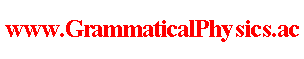since 2006 Help　Sitemap < Forum > < Products > < Quantum History Theory > < Reduced Form of Uda Equation > Yuichi Uda derived the following equation from the original form of the Uda equation on 2011/07/01.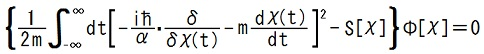where S is the action functional such that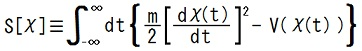. --- The derivation owes mainly to the following relation.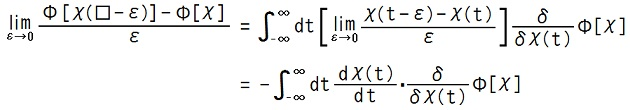I believe that this relation holds because it is analogous to the chain rule for partial differentiation. It is understood clearly by thinking of a shift of the graph of χ parallel to t-axis as a variation of χ.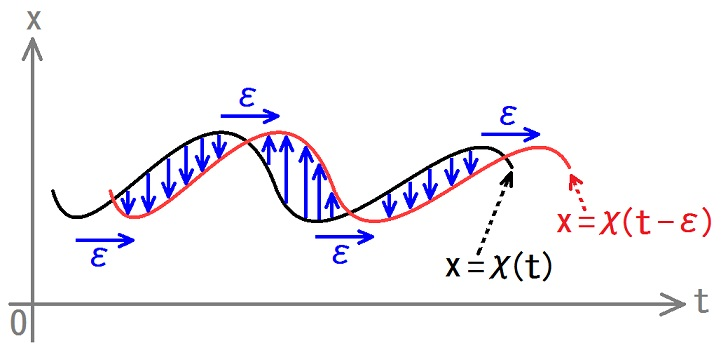Then the analogy stands as follows.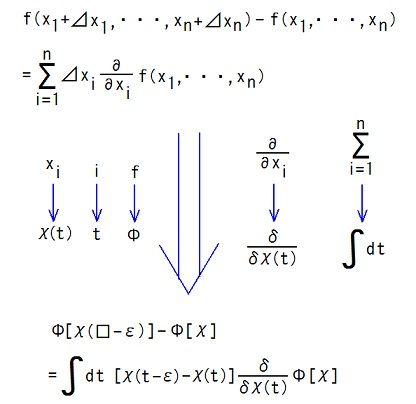In addition to it, I paid attention to the following point.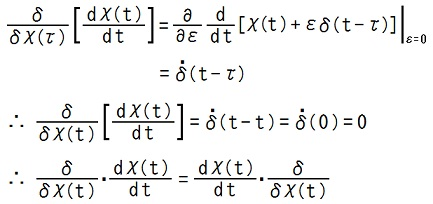Taking these two points into account, we can calculate as follows.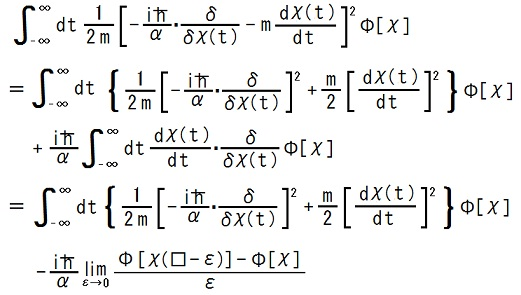By using the original form of the Uda equation here, the reduced form of it is obtained as follows.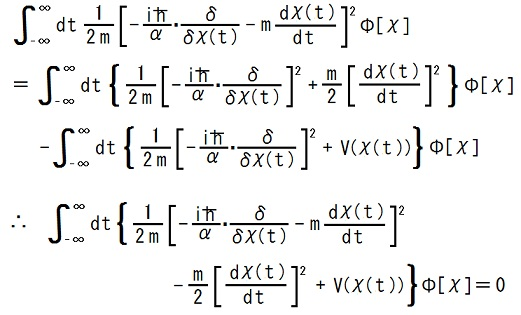--- This article is a rewrite of the article 'Reduced Form of the New Equation' in the following page. Former half@Theory of Quantum History Entangled in Time-like Direction@Products of Grammatical Physics@Grammatical Physics@Forum@Vintage(2008-2014) The content of this article was presented by me at JPS 2011 Autumn Meeting. Author Yuichi Uda, Write start at 2015/05/21/20:56JST, Last edit at 2016/04/01/13:54JST